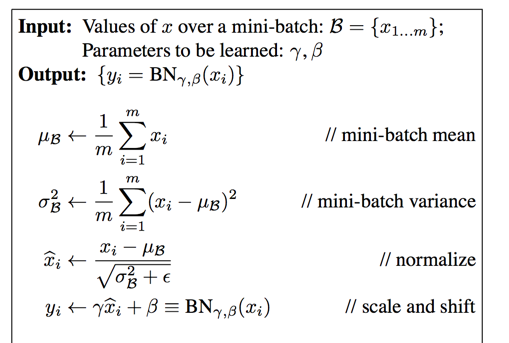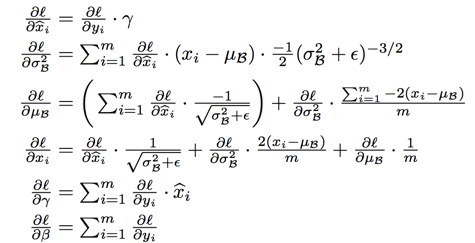Batch Normalization

Posted by GwanSiu Blog on June 6, 2017

1. Why Batch normalization?

batch normalization is normalization strategy, which makes the distribution of layers’ input consistent at the output of layers. Citate in paper: Batch normalization eliminate the effect of internal covariant shift.(What’s internal covariate shift?** The input distribution to a learning system changes during training.) Covariate shift would amplify permutation at the deeper layers. If it happens, the inputs of activation function will stay in the saturated region. In that region, the gradient will be very small and the phenomenon of vanishing gradient would happen and stop neural network to train.

Therefore, the batch normalization layer is to force the distribution of layers’ input to remain fixed over training time. Without batch normalization, sometimes the neural network used ReLU activation function still works with careful initialization and small learning rate. This is because neural network will put more effort to compensate for the changes in the distribution. Usually, it would degrade the performance of neural network.

2. The diagram of batch normalization

2.1 The Batch normalization diagram:2.2 BN train and updated3. The place of batch normalization

Generally, Batch normalization layer can apply to any input of layers. In this paper, batch normalization layers is added immediately before the activation layers. Condsider a neural network consists of an affine transformation followed by the element-wise nonlinearity:

where W and b are learned parameters of the model, and $g(·)$ is the nonlinearity such as sigmoid or ReLU.

Why not the input of the layers x? because $\mu$ is likely the output of another nonlinearity, the shape of its distribution is likely to change during training, and constraining its first and second moments would nonsense and not eliminate the covariate shift. The same principle to the convolutional layer, it means batch normalization layer is inserted between convolutional layer and the activation layer.

4. The benefits of the batch normalization

1. increase learning rate.
2. Remove the dropout and reduce the L2 weight regularization in some sense, because the batch normalization layer could regulate the neural network.
3. Accelerate the learning rate decay.
4. Remove the local response normalization.